# How to Write a Stripes Shader, Part 3: Checker Patterns

In this third part of stripes shader series we will add a new mode to render checker pattern instead stripes. We will also implement different blend operations.

The final result will look like this: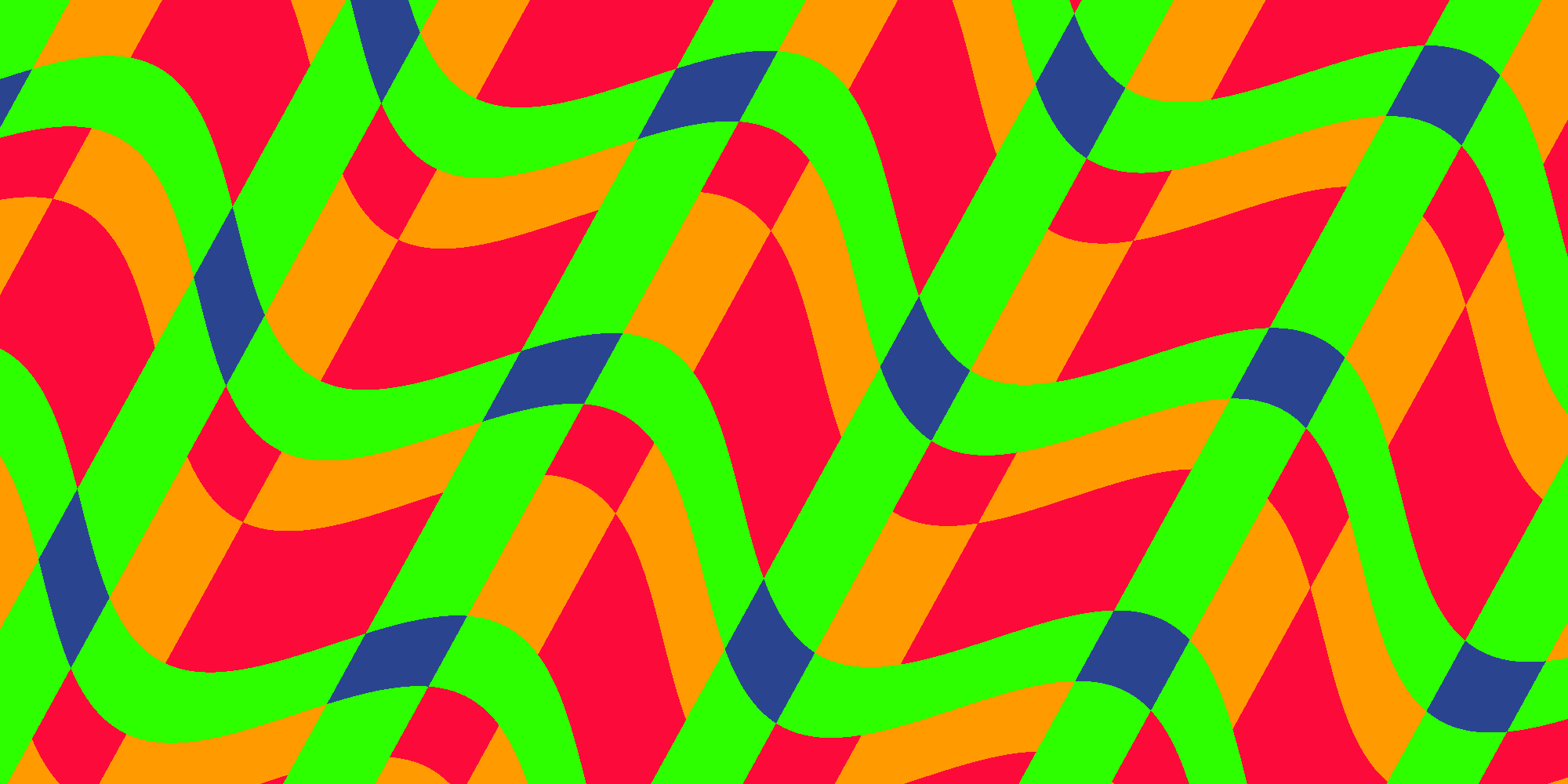## Checker Mode

Let’s start with adding a new mode in the properties. We want to select the mode from a drop down menu. This can be done by adding an `Enum` attribute to the property:

``[Enum(Stripes, 0, Checker, 1)] _Mode ("Mode", Float) = 0``

It looks like this in the Unity inspector: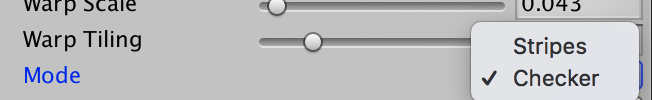Next we implement the checker mode into the fragment shader. The basic idea is to calculate `pos` and `value` in two axis. We already do this for `pos` because we need it for the warp effect. So we only need to calculate `value` in both axis, too. Then we combine the two components of `value` and use the resulting value to pick a color.

``````fixed4 frag (v2f i) : SV_Target
{
const float PI = 3.14159;

float2 pos = rotatePoint(i.uv.xy, float2(0.5, 0.5), _Direction * 2 * PI);

pos.x += sin(pos.y * _WarpTiling * PI * 2) * _WarpScale;
pos *= _Tiling;

int2 value = floor(frac(pos) * _NumColors + _WidthShift);
value = clamp(value, 0, _NumColors - 1);
if (_Mode == 1) {
value.x += value.y;
value.x = fmod(value, _NumColors);
}

switch (value.x) {
case 3: return _Color4;
case 2: return _Color3;
case 1: return _Color2;
default: return _Color1;
}
}``````

Now step by step. First we apply tiling to both components of pos:

``pos *= _Tiling;``

Then we change the type of `value` to `int2` and calculate the value from both components of `pos`:

``int2 value = floor(frac(pos) * _NumColors + _WidthShift);``

The following line did not change but since value is now an `int2` both components are being clamped. So far the result would be the same if we just render stripes.

Now comes the interesting part which actually does something different if `_Mode` equals 1 - which is the checker mode: If we are in checker mode the two components of value are being combined. Because the result can be greater than `_NumColors + 1` we perform a modulo afterwards:

``````if (_Mode == 1) {
value.x += value.y;
value.x = fmod(value, _NumColors);
}``````

In the switch we need `value.x` because value is now an `int2`:

``switch (value.x) {``

## Checker in Action

Let’s see how the new checked mode looks with two colors: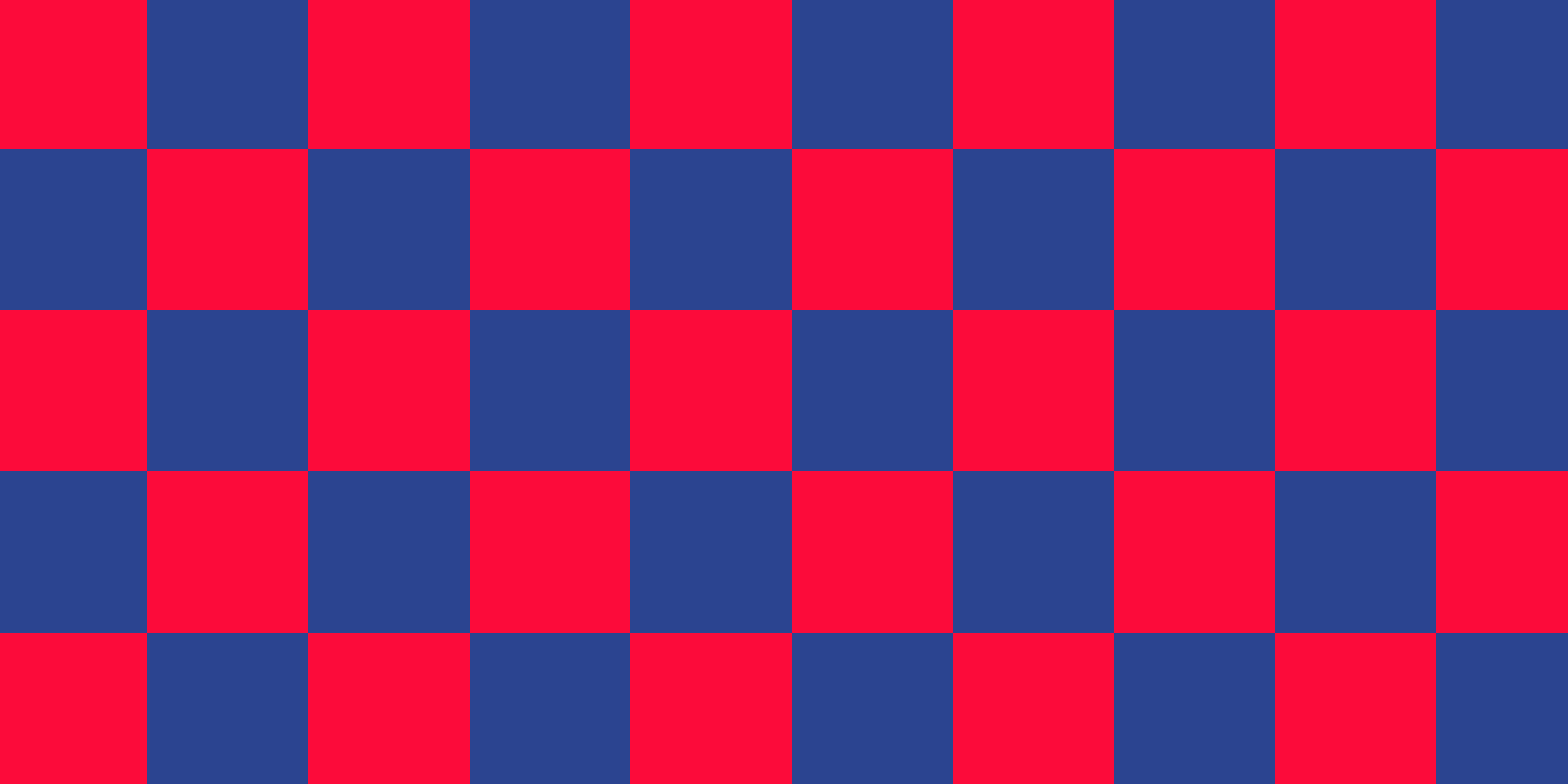Nothing fancy. But we can tweak the parameters to get more interesting results. This is a checker pattern with 4 colors: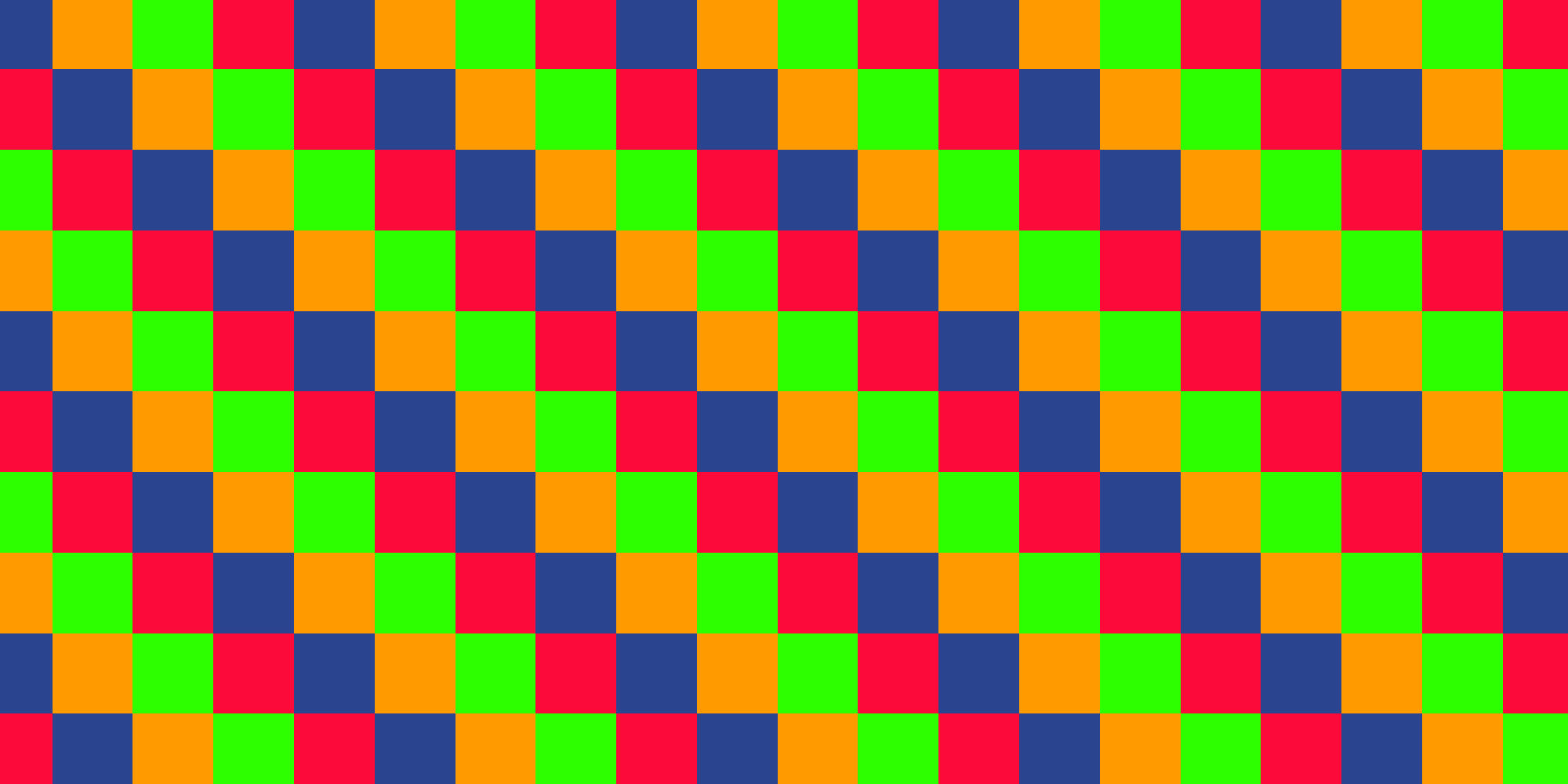The same pattern with rotation, warp and width shift parameters modified: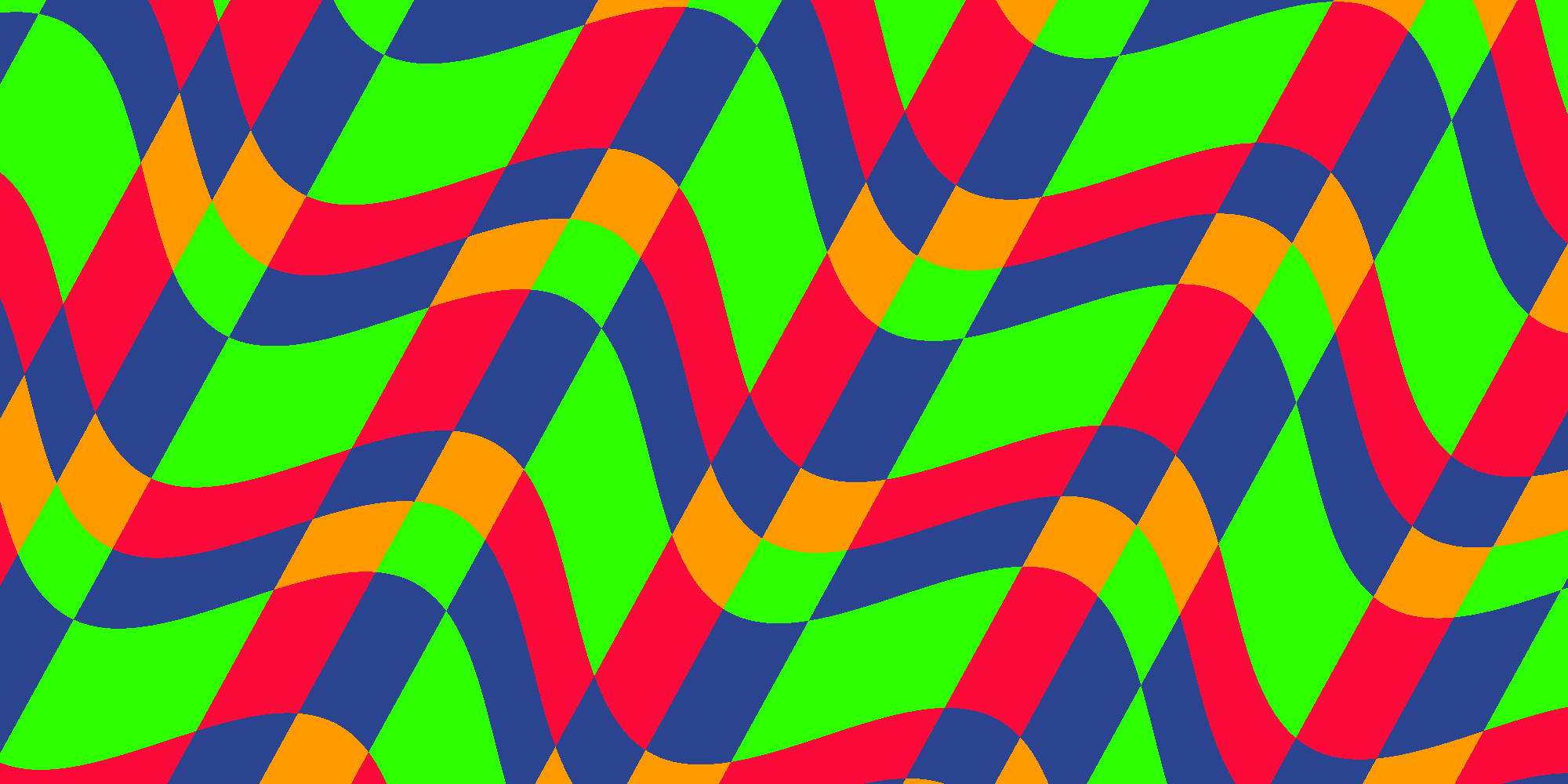## Blend Operations

So far we added the two values: `value.x += value.y`. What happens if we subtract `value.y` instead? Or use another operation like multiply, divide… We will get more variations! So let’s do that. First add another parameter:

``[Enum(Add, 0, Sub, 1, Mul, 2, Div, 3, And, 4, Or, 5)] _Operation ("Checker Operation", Float) = 0``

Then in the fragment shader switch between the different operations:

``````if (_Mode == 1) {
switch (_Operation) {
case 5:
value.x |= value.y;
break;
case 4:
value.x &= value.y;
break;
case 3:
value.x /= value.y;
break;
case 2:
value.x *= value.y;
break;
case 1:
value.x -= value.y;
break;
default:
value.x += value.y;
break;
}
value.x = fmod(value, _NumColors);
}``````Subtract: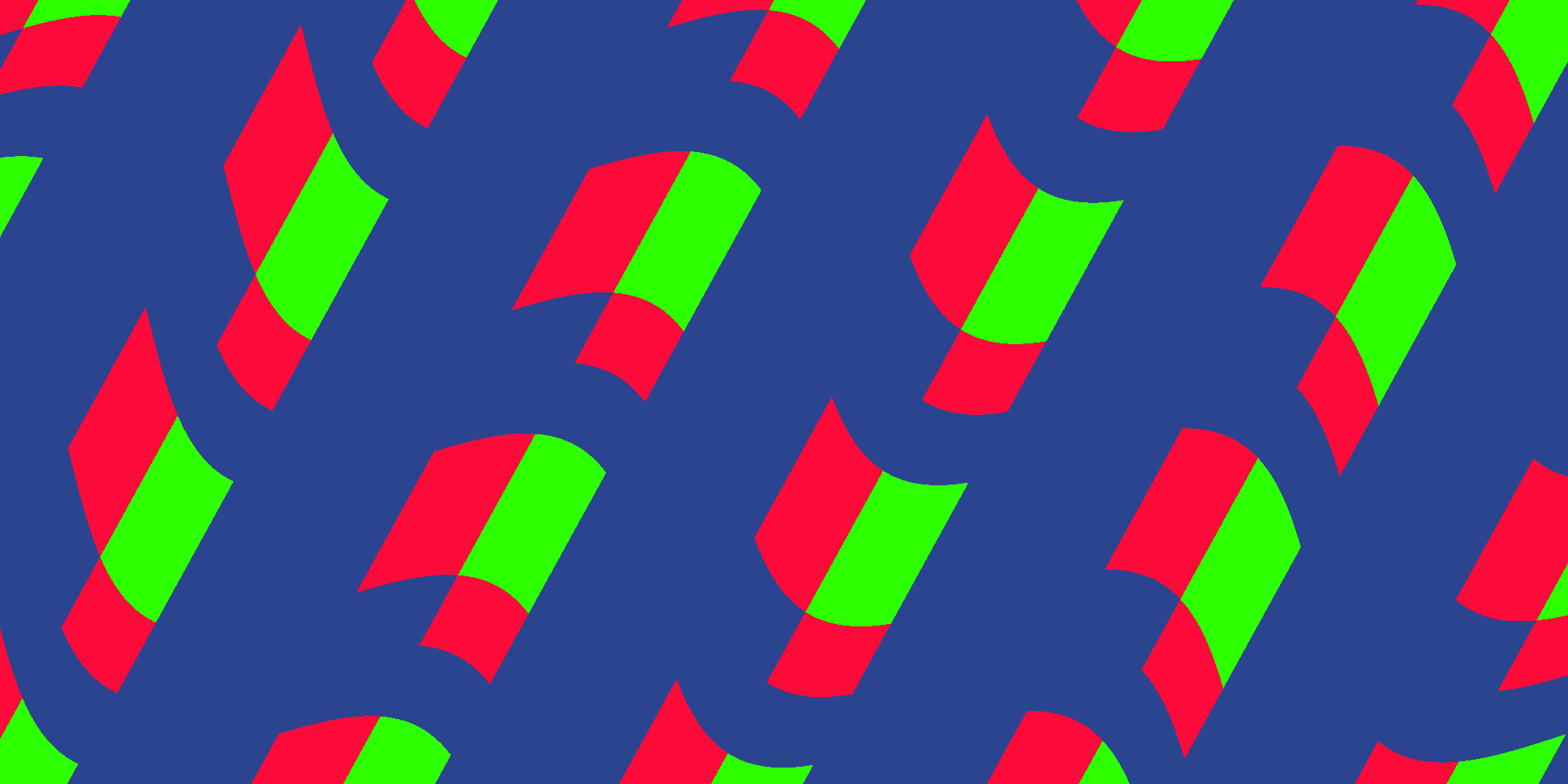Multiply:Divide: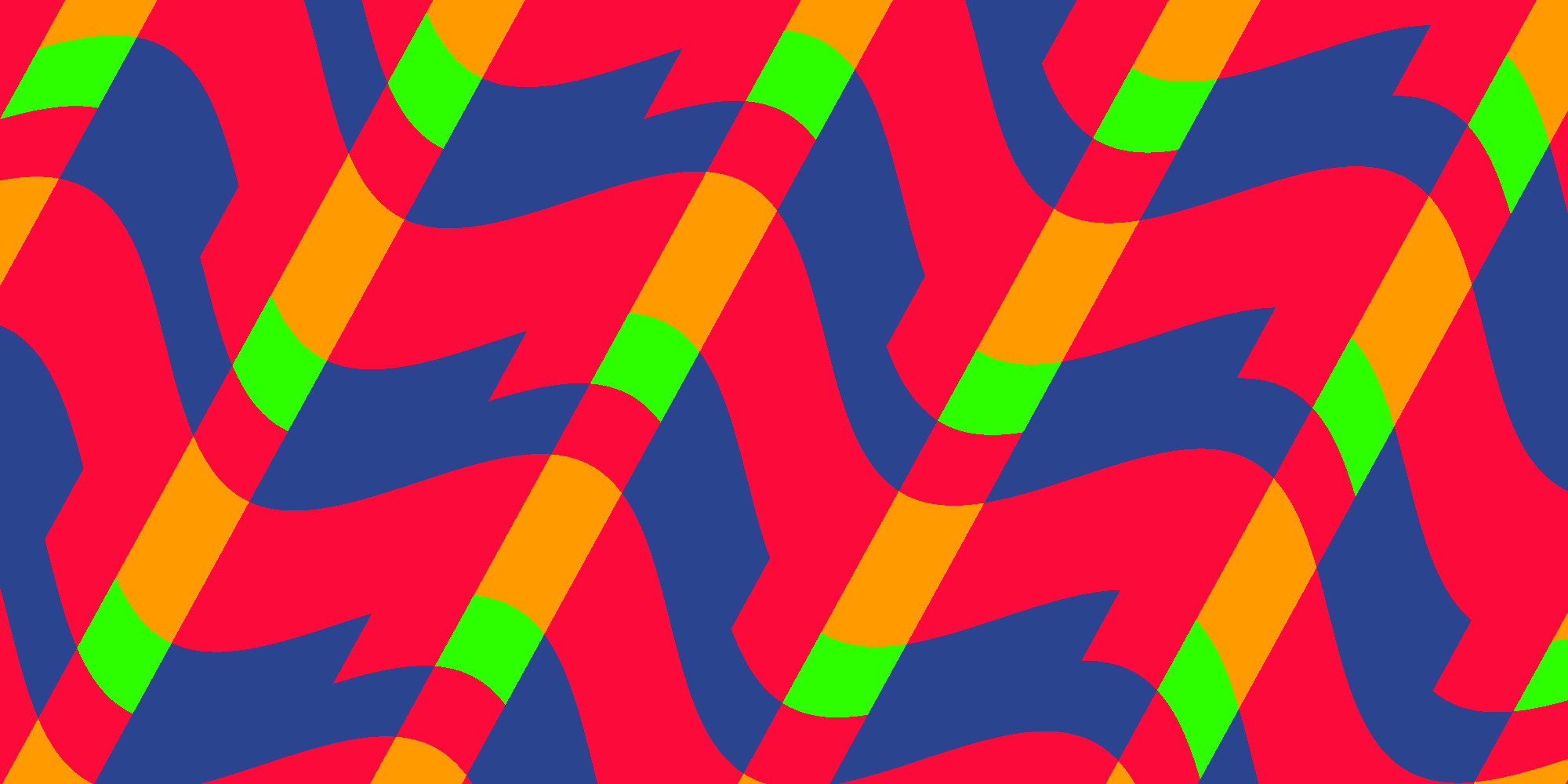Logical And: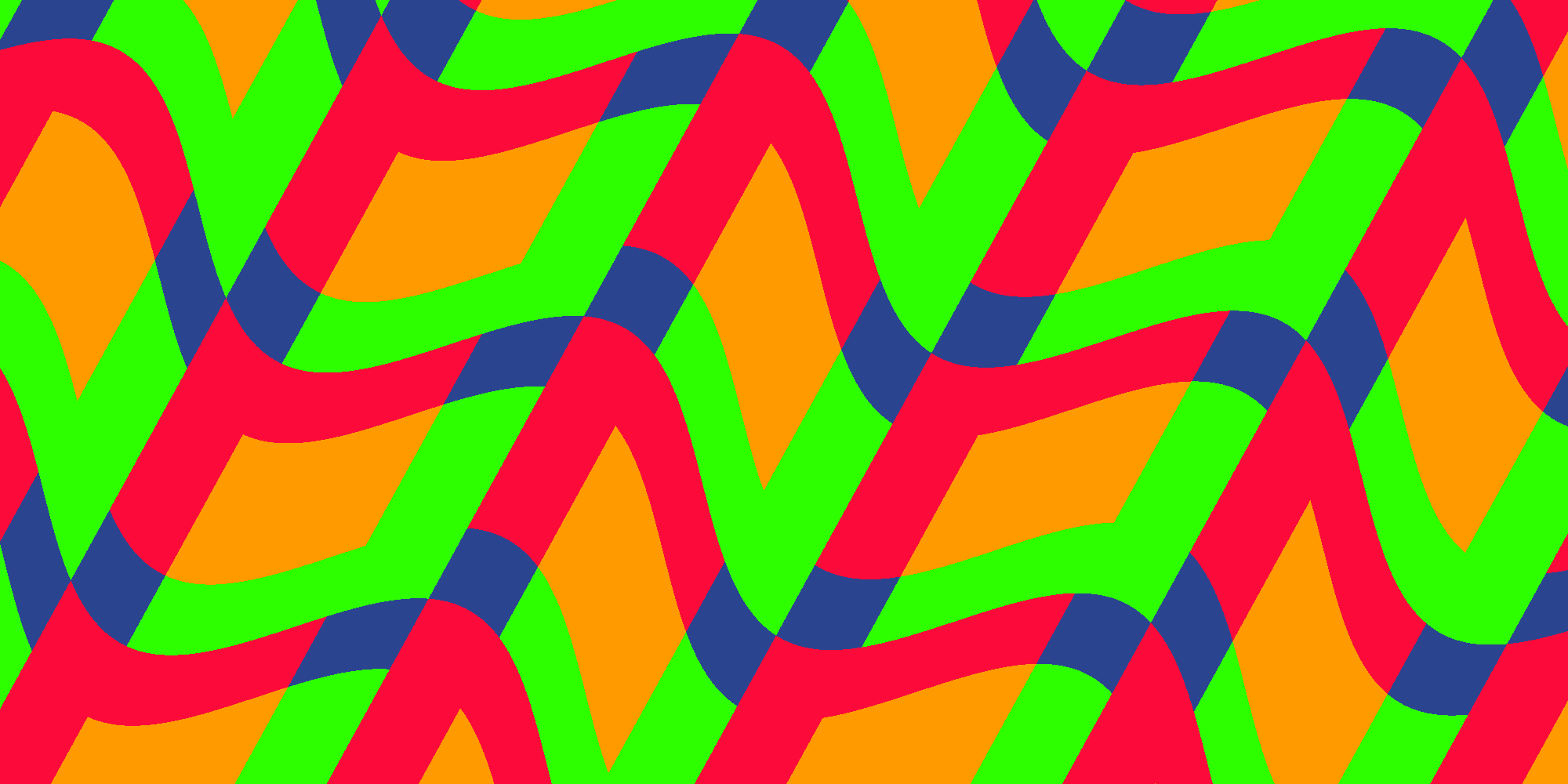Logical Or: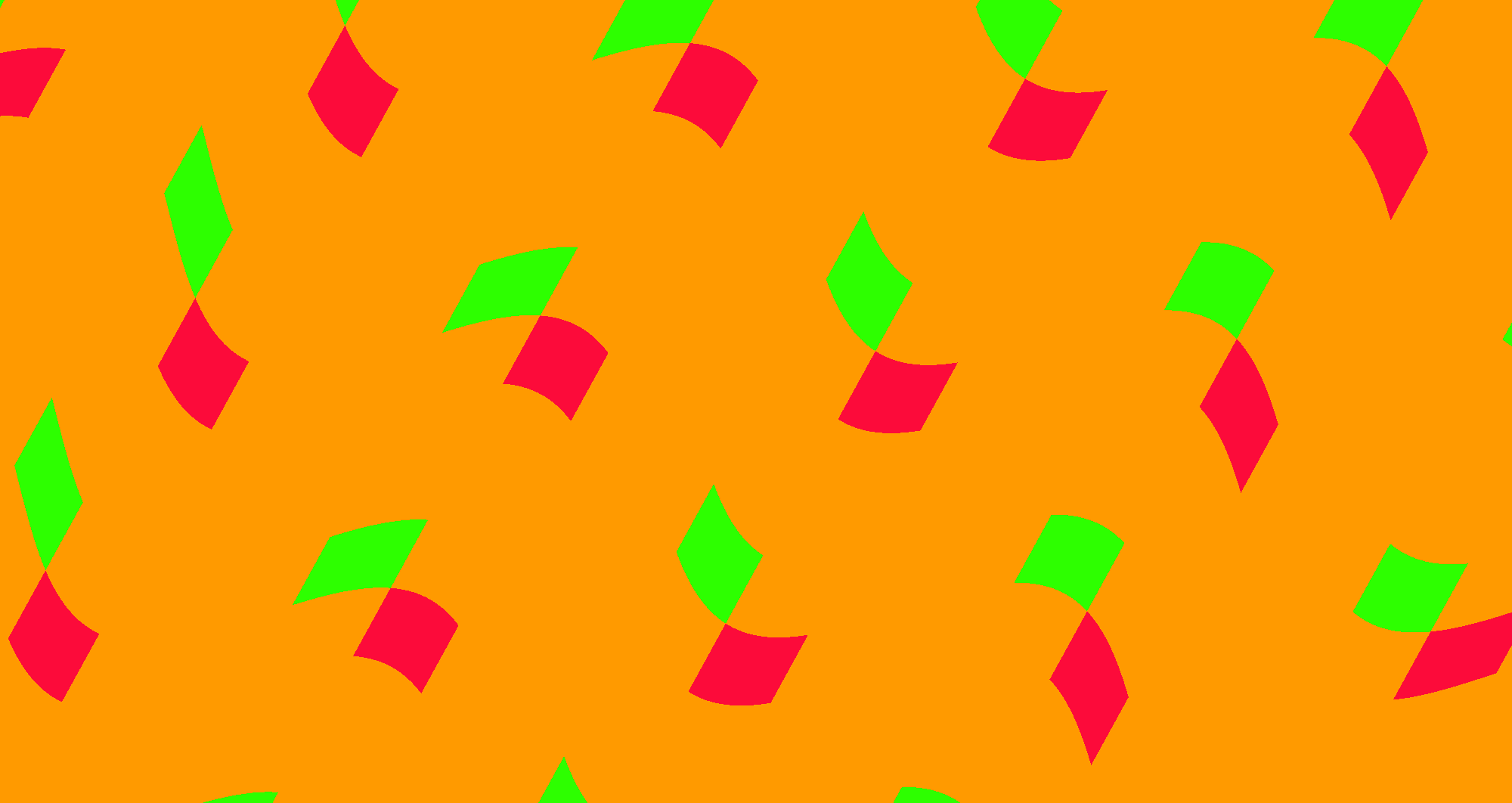## All Together

This is the full code of the shader:

``````Shader "Unlit/Stripes-3"
{
Properties {
[IntRange] _NumColors ("Number of colors", Range(2, 4)) = 2
_Color1 ("Color 1", Color) = (0,0,0,1)
_Color2 ("Color 2", Color) = (1,1,1,1)
_Color3 ("Color 3", Color) = (1,0,1,1)
_Color4 ("Color 4", Color) = (0,0,1,1)
_Tiling ("Tiling", Range(1, 500)) = 10
_WidthShift ("Width Shift", Range(-1, 1)) = 0
_Direction ("Direction", Range(0, 1)) = 0
_WarpScale ("Warp Scale", Range(0, 1)) = 0
_WarpTiling ("Warp Tiling", Range(1, 10)) = 1
[Enum(Stripes, 0, Checker, 1)] _Mode ("Mode", Float) = 0
[Enum(Add, 0, Sub, 1, Mul, 2, Div, 3, And, 4, Or, 5)] _Operation ("Checker Operation", Float) = 0
}

{

Pass
{
CGPROGRAM
#pragma vertex vert
#pragma fragment frag

#include "UnityCG.cginc"

int _NumColors;
fixed4 _Color1;
fixed4 _Color2;
fixed4 _Color3;
fixed4 _Color4;
int _Tiling;
float _WidthShift;
float _Direction;
float _WarpScale;
float _WarpTiling;
int _Operation;
int _Mode;

struct appdata
{
float4 vertex : POSITION;
float2 uv : TEXCOORD0;
};

struct v2f
{
float2 uv : TEXCOORD0;
float4 vertex : SV_POSITION;
};

v2f vert (appdata v)
{
v2f o;
o.vertex = UnityObjectToClipPos(v.vertex);
o.uv = v.uv;
return o;
}

float2 rotatePoint(float2 pt, float2 center, float angle) {
float sinAngle = sin(angle);
float cosAngle = cos(angle);
pt -= center;
float2 r;
r.x = pt.x * cosAngle - pt.y * sinAngle;
r.y = pt.x * sinAngle + pt.y * cosAngle;
r += center;
return r;
}

fixed4 frag (v2f i) : SV_Target
{
const float PI = 3.14159;

float2 pos = rotatePoint(i.uv.xy, float2(0.5, 0.5), _Direction * 2 * PI);

pos.x += sin(pos.y * _WarpTiling * PI * 2) * _WarpScale;
pos *= _Tiling;

int2 value = floor(frac(pos) * _NumColors  + _WidthShift);
value = clamp(value, 0, _NumColors - 1);
if (_Mode == 1) {
switch (_Operation) {
case 5:
value.x |= value.y;
break;
case 4:
value.x &= value.y;
break;
case 3:
value.x /= value.y;
break;
case 2:
value.x *= value.y;
break;
case 1:
value.x -= value.y;
break;
default:
value.x += value.y;
break;
}
value.x = fmod(value, _NumColors);
}

switch (value.x) {
case 3: return _Color4;
case 2: return _Color3;
case 1: return _Color2;
default: return _Color1;
}
}
ENDCG
}
}
}``````

Here you can find a Unity Package and an example scene.

Now there is a lot of parameters to play with and a lot of variation you can create with it.

This concludes the stripes shader series. I hope you enjoyed it and learned something. Also I’m curious to see what you do with it. Feel free to add your questions and feedback in the comments.